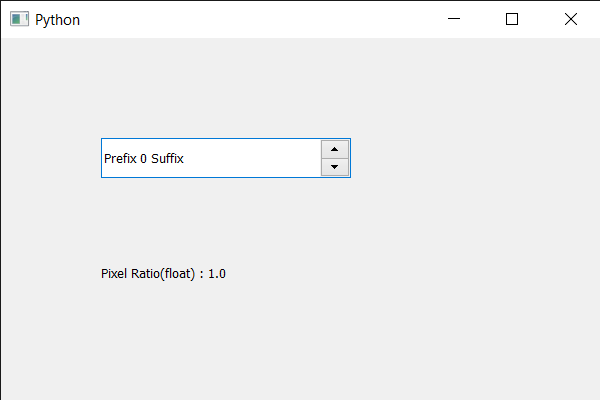# PyQt5 QSpinBox – Getting Pixel ratio as floating value

In this article we will see how we can get the pixel ratio of the spin box in the floating value, The device pixel ratio is the ratio between physical pixels and logical pixels of the spin box. Common values are 1 for normal-dpi displays and 2 for high-dpi “retina” displays. We can use `devicePixelRatio` method to get the pixel ratio but it is not in floating value.

In order to do this we use devicePixelRatioF method

Syntax : spin_box.devicePixelRatioF()

Argument : It takes no argument

Return : It returns float

Below is the implementation

 `# importing libraries ` `from` `PyQt5.QtWidgets ``import` `*`  `from` `PyQt5 ``import` `QtCore, QtGui ` `from` `PyQt5.QtGui ``import` `*`  `from` `PyQt5.QtCore ``import` `*`  `import` `sys ` ` `  ` `  `class` `Window(QMainWindow): ` ` `  `    ``def` `__init__(``self``): ` `        ``super``().__init__() ` ` `  `        ``# setting title ` `        ``self``.setWindowTitle(``"Python "``) ` ` `  `        ``# setting geometry ` `        ``self``.setGeometry(``100``, ``100``, ``600``, ``400``) ` ` `  `        ``# calling method ` `        ``self``.UiComponents() ` ` `  `        ``# showing all the widgets ` `        ``self``.show() ` ` `  `        ``# method for widgets ` `    ``def` `UiComponents(``self``): ` `        ``# creating spin box ` `        ``self``.spin ``=` `QSpinBox(``self``) ` ` `  `        ``# setting geometry to spin box ` `        ``self``.spin.setGeometry(``100``, ``100``, ``250``, ``40``) ` ` `  `        ``# setting range to the spin box ` `        ``self``.spin.setRange(``0``, ``999999``) ` ` `  `        ``# setting prefix to spin ` `        ``self``.spin.setPrefix(``"Prefix "``) ` ` `  `        ``# setting suffix to spin ` `        ``self``.spin.setSuffix(``" Suffix"``) ` ` `  `        ``# getting pixel ratio in floating value ` `        ``pixel_ratio ``=` `self``.spin.devicePixelRatioF() ` ` `  `        ``# creating label ` `        ``label ``=` `QLabel(``self``) ` ` `  `        ``# setting geometry to the label ` `        ``label.setGeometry(``100``, ``200``, ``200``, ``70``) ` ` `  `        ``# making it multi line label ` `        ``label.setWordWrap(``True``) ` ` `  `        ``# setting text to the label ` `        ``label.setText(``"Pixel Ratio(float) : "` `+` `str``(pixel_ratio)) ` ` `  `# create pyqt5 app ` `App ``=` `QApplication(sys.argv) ` ` `  `# create the instance of our Window ` `window ``=` `Window() ` `window.spin.setFocus() ` `# start the app ` `sys.exit(App.``exec``()) `

Output :My Personal Notes arrow_drop_upCheck out this Author's contributed articles.

If you like GeeksforGeeks and would like to contribute, you can also write an article using contribute.geeksforgeeks.org or mail your article to contribute@geeksforgeeks.org. See your article appearing on the GeeksforGeeks main page and help other Geeks.

Please Improve this article if you find anything incorrect by clicking on the "Improve Article" button below.

Article Tags :

Be the First to upvote.

Please write to us at contribute@geeksforgeeks.org to report any issue with the above content.